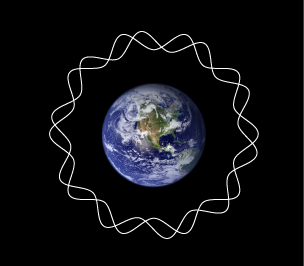# Practice: Earth's Net ChargeThe earth has a net electric charge. The resulting electric field is, on average, $150~\mbox{V/m}$ and it is directed toward the center of the earth. What is the magnitude of the Earth's net charge in Coulombs? You may assume that the charge is uniformly distributed over the Earth's surface and that the earth is a perfect sphere.

Details and assumptions

• $k=\frac{1}{4\pi \epsilon_{0}}=9\times 10^{9}~\mbox{Nm}^{2}/\mbox{C}^{2}$
• The radius of the earth is $6370~\mbox{km}$.
×

Problem Loading...

Note Loading...

Set Loading...